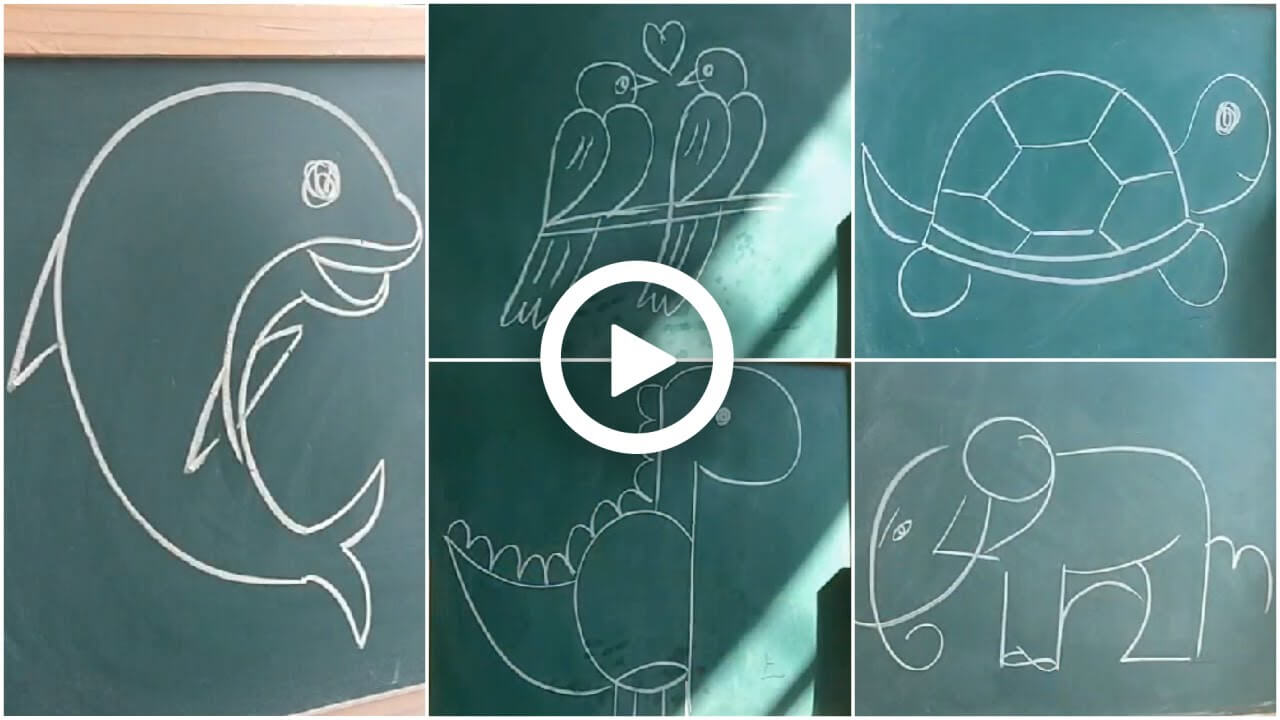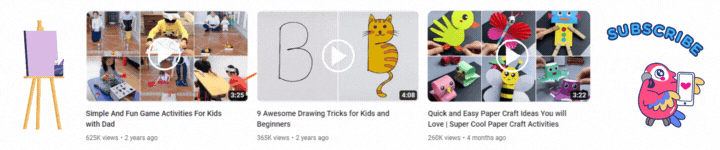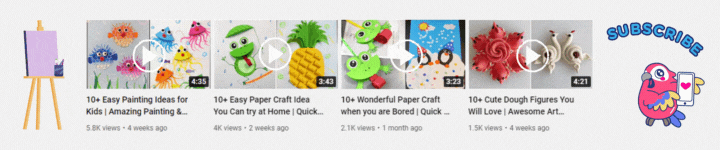Connect with us

#### Easy Animal Drawing Tricks for Kids# Easy Animal Drawing Tricks for KidsEasy and amazing drawing tricks you must try with your kids. Here are 10 simple animal drawings you should look at and guide your kids to draw. Down below are the steps that will help your kid to understand better and to draw the drawings perfectly. Your kids will have fun while drawing, so try these easy drawings and help your kids in drawing them.

## Easy Animal Drawing Tricks for Kids

Supplies:

• Blackboard/ green board
• Chalk

### 1 Drawing: Birds

Steps:

• Draw two 2’s one after the other and leaving some space draw again two 2’s.
• Now draw a semi oval at the top middle of both the 2’s, do the same with the other 2’s.
• Then draw a circle on each semi ovals and on the outside of the semi ovals draw a triangle shape.
• Now at the top of both the triangle shapes draw a heart.
• Then from the first 2 draw a line till the bottom of the 2 and then bend the line a little and extend it.
• Now draw a wavy structure at the bottom and a straight line.
• Then do the same with the other 2 as well.
• Now at the bottom of the 2’s draw a horizontal line.

### 2 Drawing: Dinosaur

Steps:

• Draw a line and at the middle of the line draw a semi oval shape.
• Then at the top of the line draw a half oval and a dot inside.
• Now from that half oval draw a straight line and merge it with the semi oval.
• Then from the backside of the semi oval draw 2 curvy lines and merge them at the end.
• Now in the inside bottom of the semi oval draw a curve.
• Then below the semi oval draw 2 vertical lines and a horizontal line and then again draw a vertical line and a horizontal line at the side.
• Now from the straight line draw curvy structures at the ending of the tail.

### 3 Drawing: Dolphin

Steps:

• Draw a C and then from the C draw a tilted 2 by curving the last line.
• Then from that point draw a small curve and then draw a less curvy C.
• Now from that draw a tilted line and a curvy V shape and a line merging the first C.
• Then from the inside of the 2 draw a curvy line and then draw a tilted V outside the first C.
• Now draw 2 tilted V’s one on the inside of the less curvy C and one on the outside.
• Then draw a circle inside the first C as eye.

### 4 Drawing: Turtle

Steps:

• Draw a semi oval and at the end make a curve.
• Now again draw a curve below that and draw 2 lines on each side and merge it with the previous curve.
• Then from the side of the semi oval draw a curvy structure as shown and draw a circle and a small curve inside it.
• Now at the bottom draw 2 semi oval shapes on each side as legs.
• Then at the side of the semi oval draw 2 curves and narrow them at the end.
• Now at the middle of the semi oval draw a hexagon shape and from the ends draw lines and merge them with the ends.

### 5 Drawing: Tree

Steps:

• Draw a curve and then at the top side draw a V shape and at the side draw a L shape.
• Now from the bottom of L draw a curve.
• Then draw a few curves on them and then from the top draw cloud shapes and merge them with the bottom curves.

### 6 Drawing: Scissor

Steps:

• Draw a X shape and then draw 2 semi circles on the bottom lines of the X.
• Now draw a small semi circle in the inside of each semi circle.
• Then on the upper lines of the X draw a curve on the outside of the line on each side.
• Now at the midpoint of the X draw a circle.

### 7 Drawing: Elephant

Steps:

• Draw a 1 and leave some space draw 2 and a tilted 3.
• Now draw a 4 by extending and curving the line of 4.
• Then at the side of the 1 draw a small ”r” and from the top line of ”r” draw a curve touching the top of 2.
• Now draw a 6 from the side of 4 and the line of 6 crossing the line of 4.
• Then draw a 7 touching the tilted 3 and draw a tilted 8 between the 1 and the ”r”.
• Now draw a 9 between the 6 and 7 and then draw 10 inside the 6 and 4.

### 8 Drawing: Fish

Steps:

• Draw a small 3 and then at the back of it draw two big 3’s.
• Then from the top and end of the small 3 draw a curve on each side and merge it with the big 3 and draw 2 lines in the middle.
• Now from the big 3 draw 2 curves from each end and merge them at the front.
• Then at the front of it draw a curve and a dot inside.
• Now outside the curve draw a small 3 as shown.

### 9 Drawing: Fox

Steps:

• Draw a triangle shape and the middle bottom of it draw a big triangle.
• Now from the side of the big triangle draw another triangle shape as the tail.
• Then at the top of the small triangle draw 2 triangles on each side as ears.
• Now at the side of the small triangle draw a dot and 3 lines on each side.
• Then inside the small triangle draw 2 curvy lines as eyes.
• Now draw 2 triangle shapes on the inside bottom of the big triangle.

### 10 Drawing: Puppies

Steps:

• Draw a  tilted 6 and then from the same line draw another 6 with an oval shape at the end.
• Now draw a curve at the bottom of each 6.
• Then inside one six draw 2 oval shapes as eyes and then below that a dot and then a tilted 3.
• Now on the other 6 draw 2 curves as eyes and then a dot and then a tilted 3.
• Then at the below of the first 6 draw a curvy W and do the same with the other 6.
• Now inside the curvy W’s draw an oval at the middle of each and 2 curvy lines on each side of the oval.

You are done with all 10 animal drawings. Easy and simple drawing your kids must try. These will help them to learn more and as well as they will enjoy it. So engage your kids in these drawing activities and let them have fun.You may also like...
Click to comment